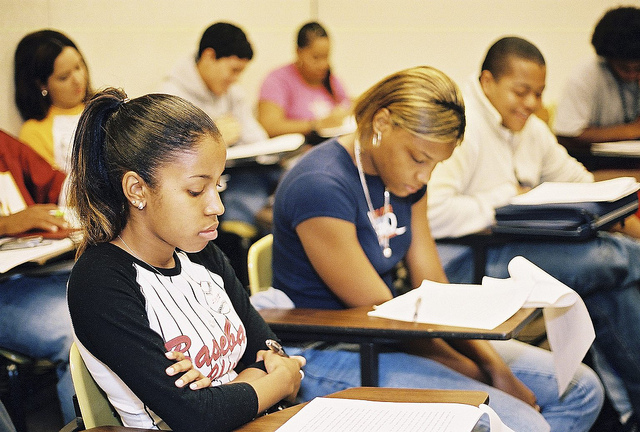## Putting It Together: Multi-Step Linear Equations

Kristy, the college student we met earlier who was anxiously studying for her final exam, still isn’t sure what grade she needs to earn to pass her math class, and the final is tomorrow! Let’s put your new math skills to good use to help her figure it out.

• Kristy’s current grade in the class is $82.3$%.
• She wants to know what score she needs on the final to keep her B in the class ($\ge80\%$).
• She also wants to know what score she needs on the final to pass the class with a C ($\ge70\%$).Exam time is coming.

Even though Kristy knows her current grade is $82.3$%, that isn’t enough information to determine what grade she needs on the final. She also needs to know how much the final exam can affect her grade. To figure this out, she consults her class syllabus, where she finds the following breakdown of points in the class:

 Homework 150 Points Quizzes 100 Points Midterm 100 Points Final Exam 200 Points TOTAL 550 Points

How can we put this information together in a way that will be useful?

First, we know that Kristy has earned points for everything but the final exam. If we add up the points for homework, quizzes, and the midterm, we know there are $350$ points possible so far ($150+100+100$). If Kristy’s grade is currently $82.3$%, this means she has earned $82.3$% of the total $350$ points. We can multiply to find the number of points she has earned so far:

$350 \times 0.823 = 288.05$ points

So we know that Kristy has $288.05$ points. The next question to answer is: how many total points does she need to achieve a B or a C?

Typically a B is a total score of $80$% or $.8$, and a C is $70$% or $.7$

There are a total of $550$ points in the class. We can use this to figure out how many points Kristy would need to get a certain grade:

To get a B, she needs $550 \times 0.8 = 440$ points.

To get a C, she needs $550 \times 0.7 = 385$ points.

Now we can write a linear equation to determine the number of points she needs on the final to earn either a B or a C in the class.

current number of points + points needed on the final = total points needed

Again, Kristy has $288.05$ points. Let’s call the number of points she needs on the final $x$. We know that for a B, she needs $440$ points total. We can set up the equation and solve for $x$ to determine the number of points she needs on the final to earn a B.

$288.05+x=440$

$288.05+x\color{red}{-288.05}=440\color{red}{-288.05}$

$x=151.95$

To state that grade as a percentage, we can divide it by the total number of points possible on the final exam: $200$. That means Kristy needs $151.95/200=75.975$%. In other words, a C grade of $76$% on the final will be enough for her to get a B in the class.

Now let’s calculate how many points she can earn on the final to pass the class with a C. We’ll use the same equation, but this time, we’ll use $385$ for the total number of points.

$288.05 + x = 385$

Solve again, and you’ll find that $x = 96.95$. If we divide that by $200$ to figure out the percentage, we learn that Kristy needs a score of $48.475$% on the final to get a C in the class. In other words, she can fail the final exam earning a $49$% and still pass the class.

Kristy can now take the final exam knowing exactly how well she needs to do to pass the class and keep her scholarship–and it turns out that she doesn’t need to be as nervous as she thought!